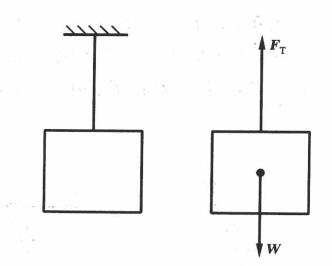# 物体的受力平衡

2020年7月17日19:39:48 发表评论浏览：262

### 1.共线力系的平衡

∑F=0

∑MA(F)=0### 2平面力系的平衡

∑F=0

∑Fx=0，∑Fy=0

∑Mo(F)=0

∑Fx=0，∑Fy=0，∑Mo(F)=0

### 3空间力系的平衡

∑Fx=0，∑Fy=0，∑Fz=0

∑Mz(F)=0，∑My(F)=0，∑Mz(F)=0

∑Fx=0，∑Fy=0，∑Fz=0

∑Mz(F)=0，∑My(F)=0，∑Mz(F)=0